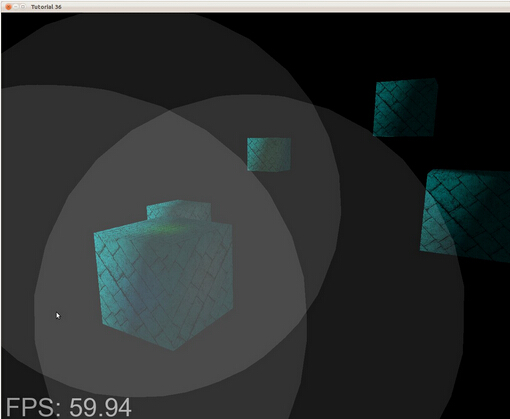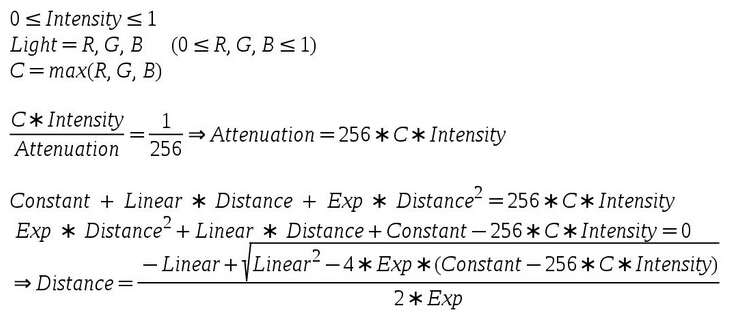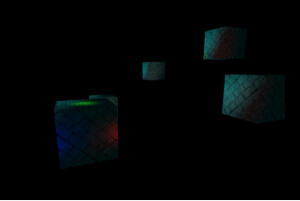modern opengl tutorial

end

# 第三十六课 延迟渲染（二）

## 背景## 代码

``````(tutorial36.cpp:142)
virtual void RenderSceneCB()
{
CalcFPS();
m_scale += 0.05f;
m_pGameCamera->OnRender();
DSGeometryPass();
BeginLightPasses();
DSPointLightsPass();
DSDirectionalLightPass();
RenderFPS();
glutSwapBuffers();
} ``````

``````(tutorial36.cpp:164)
void DSGeometryPass()
{
m_DSGeomPassTech.Enable();
m_gbuffer.BindForWriting();
// Only the geometry pass updates the depth buffer
glClear(GL_COLOR_BUFFER_BIT | GL_DEPTH_BUFFER_BIT);
glEnable(GL_DEPTH_TEST);
glDisable(GL_BLEND);
Pipeline p;
p.SetCamera(m_pGameCamera->GetPos(), m_pGameCamera->GetTarget(), m_pGameCamera->GetUp());
p.SetPerspectiveProj(m_persProjInfo);
p.Rotate(0.0f, m_scale, 0.0f);
for (unsigned int i = 0 ; i < ARRAY_SIZE_IN_ELEMENTS(m_boxPositions) ; i++) {
p.WorldPos(m_boxPositions[i]);
m_DSGeomPassTech.SetWVP(p.GetWVPTrans());
m_DSGeomPassTech.SetWorldMatrix(p.GetWorldTrans());
m_box.Render();
}
// When we get here the depth buffer is already populated and the stencil pass
// depends on it, but it does not write to it.
glDisable(GL_DEPTH_TEST);
} ``````

``````(tutorial36.cpp:199)
void BeginLightPasses()
{
glEnable(GL_BLEND);
glBlendFunc(GL_ONE, GL_ONE);
glClear(GL_COLOR_BUFFER_BIT);
}``````

``````(tutorial36.cpp:210)
void DSPointLightsPass()
{
m_DSPointLightPassTech.Enable();
m_DSPointLightPassTech.SetEyeWorldPos(m_pGameCamera->GetPos());
Pipeline p;
p.SetCamera(m_pGameCamera->GetPos(), m_pGameCamera->GetTarget(), m_pGameCamera->GetUp());
p.SetPerspectiveProj(m_persProjInfo);
for (unsigned int i = 0 ; i < ARRAY_SIZE_IN_ELEMENTS(m_pointLight); i++) {
m_DSPointLightPassTech.SetPointLight(m_pointLight[i]);
p.WorldPos(m_pointLight[i].Position);
float BSphereScale = CalcPointLightBSphere(m_pointLight[i]);
p.Scale(BSphereScale, BSphereScale, BSphereScale);
m_DSPointLightPassTech.SetWVP(p.GetWVPTrans());
m_bsphere.Render();
}
} ``````

``````(tutorial36.cpp:275)
float CalcPointLightBSphere(const PointLight& Light)
{
float MaxChannel = fmax(fmax(Light.Color.x, Light.Color.y), Light.Color.z);
float ret = (-Light.Attenuation.Linear + sqrtf(Light.Attenuation.Linear * Light.Attenuation.Linear -
4 * Light.Attenuation.Exp * (Light.Attenuation.Exp - 256 * MaxChannel * Light.DiffuseIntensity)))
/
2 * Light.Attenuation.Exp;
return ret;
} ``````

``````(tutorial36.cpp:230)
void DSDirectionalLightPass()
{
m_DSDirLightPassTech.Enable();
m_DSDirLightPassTech.SetEyeWorldPos(m_pGameCamera->GetPos());
Matrix4f WVP;
WVP.InitIdentity();
m_DSDirLightPassTech.SetWVP(WVP);
} ``````

``````(light_pass.vs)
#version 330
layout (location = 0) in vec3 Position;
uniform mat4 gWVP;
void main()
{
gl_Position = gWVP * vec4(Position, 1.0);
} ``````

``````(dir_light_pass.fs:108)
void main()
{
vec2 TexCoord = CalcTexCoord();
vec3 WorldPos = texture(gPositionMap, TexCoord).xyz;
vec3 Color = texture(gColorMap, TexCoord).xyz;
vec3 Normal = texture(gNormalMap, TexCoord).xyz;
Normal = normalize(Normal);
FragColor = vec4(Color, 1.0) * CalcDirectionalLight(WorldPos, Normal);
}
(point_light_pass.fs:109)
void main()
{
vec2 TexCoord = CalcTexCoord();
vec3 WorldPos = texture(gPositionMap, TexCoord).xyz;
vec3 Color = texture(gColorMap, TexCoord).xyz;
vec3 Normal = texture(gNormalMap, TexCoord).xyz;
Normal = normalize(Normal);
FragColor = vec4(Color, 1.0) * CalcPointLight(WorldPos, Normal);
} ``````

``````(dir_light_pass.fs:101, point_light_pass.fs:101)
vec2 CalcTexCoord()
{
return gl_FragCoord.xy / gScreenSize;
} ``````

``````(gbuffer.cpp:49)
bool GBuffer::Init(unsigned int WindowWidth, unsigned int WindowHeight)
{
...
for (unsigned int i = 0 ; i < ARRAY_SIZE_IN_ELEMENTS(m_textures) ; i++) {
...
glTexParameterf(GL_TEXTURE_2D, GL_TEXTURE_MIN_FILTER, GL_NEAREST);
glTexParameterf(GL_TEXTURE_2D, GL_TEXTURE_MAG_FILTER, GL_NEAREST);
...
}
...
} ``````

``````(gbuffer.cpp:98)
{
glBindFramebuffer(GL_DRAW_FRAMEBUFFER, 0);
for (unsigned int i = 0 ; i < ARRAY_SIZE_IN_ELEMENTS(m_textures); i++) {
glActiveTexture(GL_TEXTURE0 + i);
glBindTexture(GL_TEXTURE_2D, m_textures[GBUFFER_TEXTURE_TYPE_POSITION + i]);
}
} ``````

BUG，BUG....

## 操作结果